Judgments

foundations

# Judgments

## Idea

In mathematics and especially in formal logic, by a judgement [Frege (1879, §2)] one means the assertion [Russell & Whitehead (1910, p. xviii)] or validation [Kochen (1961, p. 2)] of a proposition $P$, hence the claim (or even the specification) of a proof of $P$ [Church (1940, §5)].

To bring out the subtle (cf. jstor:43154266) but crucial distinction — eloquently amplified by Martin-Löf (1996, lectures 1-2) — between the notion of a proposition $P$ as such and the judgement/assertion/validation/proof of its truth, it is tradition to prefix the latter with the symbol “$\vdash$”, known as the Urteilsstrich (German: “judgement stroke”) of [Frege (1879, §2)] (see the historical pointers below), as:

(1)$\vdash \; P \,.$

For example, the Riemann hypothesis certainly is a proposition, but it remains open whether we may truthfully assert or validate it, hence we cannot yet judge that it holds true: No proof is known, even though it seems likely to be in fact true.

In other words, the judgement symbols “$\vdash \, P$ ” convey that we know (a proof of, hence the truth of) $P$ [Martin-Löf (1996, lectures 1-2)].

As such, judgements may be formalized as “meta-proposition”, namely propositions but in an ambient meta-language (the deductive system or logical framework) rather than to the object language in which the given $P$ itself is formulated. (More specifically, any deductive system includes, as part of its specification, which strings of symbols are to be regarded as the judgments. Some of these symbols may themselves express a proposition in the object language, but this is not necessarily the case.)

More generally, one considers hypothetical judgements (maybe first made explicit in [Church (1940, §5)]) which are such assertions/validations but conditioned on premises, hence claims/specifications of proofs based on assumptions $A$: Writing

(2)$A \;\;\vdash\;\; P$

is to express the judgement that given any assertion/validation/proof of $A$ we know how to produce (from that) a validation/proof of $P$.

In systems of (dependent) type theory (where such notation seems to go back to Hofmann (1995, p. 31), cf. below) this is quite literally the case in that the categorical semantics of such a hypothetical judgement is a given by a function (and typically a computable function, hence an algorithm) which maps proofs of $A$ to proofs of $P$.

More generally yet, in type theory the propositions $A$ and $P$ appearing here are allowed to be any (dependent) types (see at propositions as types). In this generality, the denotational semantics of hypothetical judgements (2) is given by (computable) functions/morphisms with domain $A$ and codomain $P$. In programming languages based on such type theories this translates to the denotation of an actual program which produces data of data type $P$ when run on input data of data type $A$.

Judgements are themselves subject to a calculus of natural deduction by which further judgements may be inferred from given ones. For example the notation

$\frac{ A \;\;\vdash\;\; P_1 ,\, \;\;\;\;\;\;\; A \;\;\vdash\;\; P_1 }{ A \;\;\vdash\;\; P_1 \times P_2 }$

traditionally expresses that whenever we can assert both $P_1$ as well as $P_2$ assuming a validation of $A$ (hence: given a pair of functions with domain type $A$), then with that same assumption we find assertion/validation/proof of their logical conjunction $P_1 \times P_2$ (namely a function with codomain to their product type).

Finally, to make explicit which particular assumptions a hypothetical judgement really depends on, it is common to adjoin a generic context symbol $\Gamma$ to the hypothesis on the left hand side of the Urteilsstrich: Notation such as

$\Gamma ,\,\;\; a \colon A \;\;\; \vdash \;\;\; p_a \,\colon\, P$

expresses the judgement that $P$ may be asserted given validation of $\Gamma \times A$, but that in doing so only the validation of $A$ is actually made use of, while $\Gamma$ may be any context of assumptions (whose validation might however be used in previous/further deduction steps).

## Examples

### In first-order logic

In first-order logic, a paradigmatic example of a judgement is the judgement that a certain string of symbols is a well-formed proposition. This is often written as “$P \;prop$”, where $P$ is a metavariable standing for a string of symbols that denotes a proposition.

Another example of a judgement is the judgement that these symbols form a proposition proved to be true. This judgment is often written as “$P\;true$”.

Neither of these judgements is the same thing as the proposition $P$ itself. In particular, the proposition is a statement in the logic, while the judgement that the proposition is a proposition, or is provably true, is a statement about the logic. However, often people abuse notation and conflate a proposition with the judgment that it is true, writing $P$ instead of $P\;true$.

### In type theory

The distinction between judgements and propositions is particularly important in intensional type theory.

The paradigmatic example of a judgment in type theory is a typing judgment. The assertion that a term $t$ has type $A$ (written “$t:A$”) is not a statement in the type theory (that is, not something which one could apply logical operators to in the type-theoretic system) but a statement about the type theory.

Often, type theories include only a particular small set of judgments, such as:

• typing judgments (written $t:A$, as above)

• judgments of typehood (usually written $A \;type$)

• judgments of equality between typed terms (written say $(t=t'):A$)

(In a type theory with a type of types, judgments of typehood can sometimes be incorporated as a special case of typing judgments, writing $A:Type$ instead of $A\;type$.)

These limited sets of judgments are often defined inductively by giving type formation/term introduction/term elimination- and computation rules (see natural deduction) that specify under what hypotheses one is allowed to conclude the given judgment.

These inductive definitions can be formalized by choosing a particular type theory to be the meta-language; usually a very simple type theory suffices (such as a dependent type theory with only dependent product types). Such a meta-type-theory is often called a logical framework.

## Hypothetical and generic judgments

It may happen that a judgment $J$ is only derivable under the assumptions of certain other judgments $J_1,\dots, J_2$. In this case one writes

$J_1,\dots,J_n \;\vdash J.$

Often, however, it is convenient to incorporate hypotheticality into judgments themselves, so that $J_1,\dots,J_n \;\vdash J$ becomes a single hypothetical judgment. It can then be a consequence of other judgments, or (more importantly) a hypothesis used in concluding other judgments. For instance, in order to conclude the truth of an implication $\phi\Rightarrow\psi$, we must conclude $\psi$ assuming $\phi$; thus the introduction rule for implication is

$\frac{\phi \;\vdash\; \psi}{\vdash\; \phi\Rightarrow\psi}$

with a hypothetical judgment as its hypothesis. See natural deduction for a more extensive discussion.

In a type theory, we may also consider the case where the hypotheses $J_1$ are typing judgments of the form $x:A$, where $x$ is a variable, and in which the conclusion judgment $J$ involves these variables as free variables. For instance, $J$ could be $\phi\;prop$, where $\phi$ is a valid (well-formed) proposition only when $x$ has a specific type $X$. In this case we have a generic judgement, written

$(x \colon X) \;\vdash\; (\phi \; prop).$

which expresses that assuming the hypothesis or antecedent judgement that $x$ is of type $X$, as a consequence we have the succedent judgement that $\phi$ is a proposition. If on the right here we have a typing judgment

$(x \colon X) \;\vdash\; (t \colon A)$

we have a term in context.

For more about the precise relationship between the various meanings of $\vdash$ here, see natural deduction and logical framework.

While this may seem to be a very basic form of (hypothetical/generic) judgement only, in systems such as dependent type theory or homotopy type theory, all of logic and a good bit more is all based on just this.

## History of the notion and notation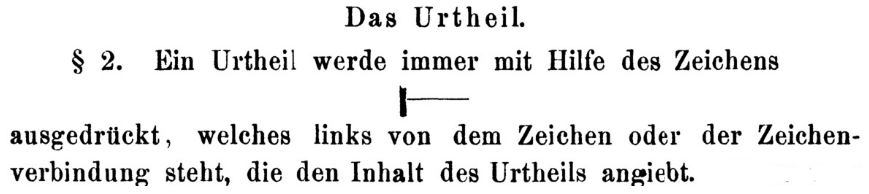The terminology of judgement in formal logic, and the notation

$\vdash A$

for the judgement of content $A$ is due to Frege (1879, §2) (Frege’s das Urtheil – or das Urteil in modern German spelling – directly translates to: the judgement).

This notion and notation (often “Frege’s Urteilsstrich” – “judgement stroke”) was adopted by Russell & Whitehead (1910, p. xviii), who however speak of “assertion” instead of “judgement” — see Martin-Löf (1996, p. 6) for speculation that these authors may have wanted “to avoid any association with Kantian philosophy” (cf. below):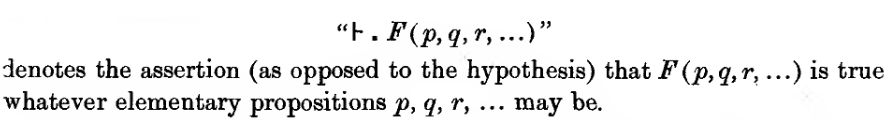and then by Church (1940, §5) (without any attribution) — now allowing hypotheses/assumptions on the left of the Urteilsstrich — to mean provable under these assumptions: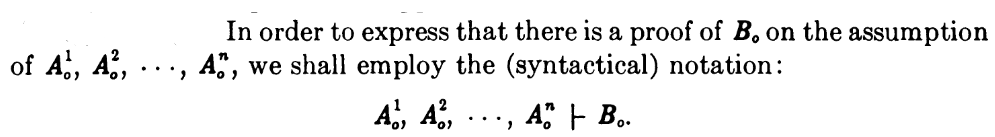Similarly, Kochen (1961, p. 2) introduced (in a context of model theory) the notation “$\mathfrak{A} \;\vDash\; P$” (apparently not actively remembering its historical origin, cf. Kochen (2017)) for a notion in model theory pronounced “$\mathfrak{A}$ validates $P$” — which is at least closely related to these hypothetical judgements (and often used synonymously, e.g. here):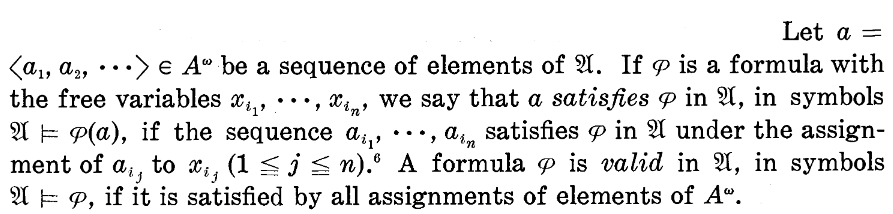The need (which had been contested by some logicians such as Wittgenstein, cf. jstor:43154266) for Frege’s distinction between a proposition “$A$”, as such, and its judgement/assertion/proof/validation “$\vdash A$” was eloquently argued for by Martin-Löf (1984, pp. 2), (1987) and in (1996, lectures 1-2), together with a re-promotion of Frege’s Urteilsstrich notation “$\vdash$” (ML84, p. 2, ML96, p. 2, 6):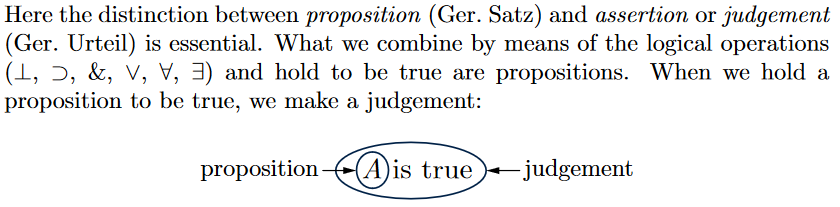The notation for hypothetical judgements

(3)$context \;\vdash\; judgement$

was then (without attribution to Church or Martin-Löf) adopted for dependent type theory in Hofmann (1995, p. 31), later published as Hofmann (1997, p. 82):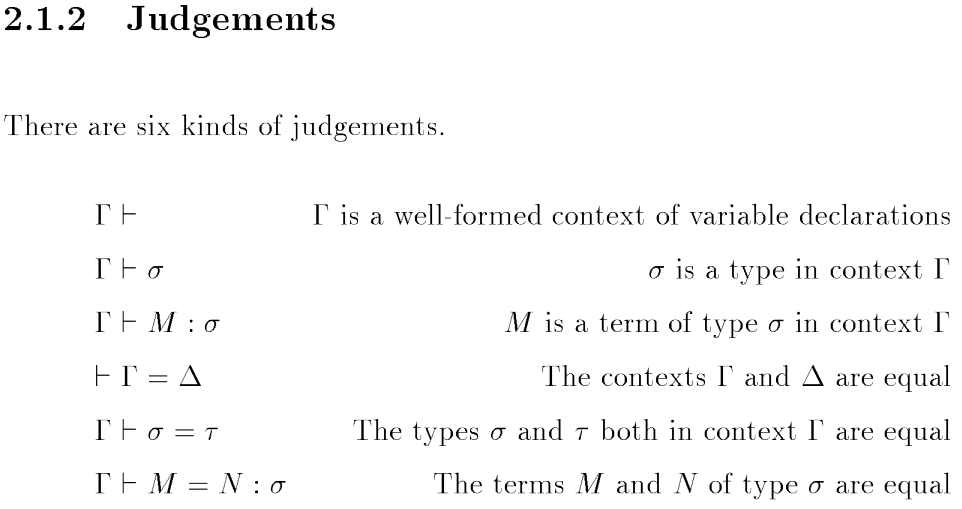This eventually became the trademark of the practice of dependent type theory (though mostly used without any attribution), e.g.: Jacobs (1998, p. 2, 121, 586); Bauer, Haselwarter & Lumsdaine (2020).

(Curiously, apparently Martin-Löf did not actually use the general notation (3) in publications: When in 1996, p. 29 it comes to hypothetical judgements, he switched to using just a vertical “$\vert$” instead of “$\vdash$”.)

In summary:

terminologyauthor
judgementFrege (1879)
proofChurch (1940)
validationKochen (1961)
judgementMartin-Löf (1984, 1996)
Hofmann (1995, 1997)

mathematical statements

Pre-history of the notion of judgement (Urteil) in (informal) logic:

The origin of the notion of “judgement” (and of the notation “$\vdash$” for it) in formal logic:

and under the name “assertion” in:

Keeping the symbol “$\vdash$”, now allowing hypothesis on the left, but pronouning it as “proof under assumptions”:

Discussion in the context of what became known as Martin-Löf dependent type theory:

Reviewed in:

Further discussion in

Textbook acccount in a context of programming languages:

The alternative notation “$\vDash$” for satisfaction with closely related (if different) usage seems to originate in

whose author

cannot recollect whether [he] originated it or took it from some other paper.

according to: